# Introduction

We are able to drive a DC motor using a transistor.. In one direction. But what if you want to be able to drive it in two different directions - both forward AND back - how do we do that? Let me introduce the H-bridge!

So for propulsion in both directions, we take our DC motor and connect it to our H-Bridge in a way so that the current can flow through the module in both directions. Perhaps easier said than done, but this is the concept that allows the DC motor to be able to operate in both directions.

This blog will attempt to explain the functionality of an H-bridge and then we will go through a demonstration of the setup of the circuit. This demonstration will include a code and a reference will be made to the code used. If you are only interested in the implementation and not the actual theory of an H-bridge, click here!

The H-bridge is therefore a very useful tool and is used in many technologies. But what is an H-bridge and how does it work? An H-bridge can actually consist of a set of transistors (or MOSFET's) working together in a network. This network typically consists of four transistors. The direction of the current through the network depends on the logic values ​​(HIGH/LOW or 1 and 0) of the various pins in the network. And these logic values ​​are dictated by the voltage that exists across the bases of the transistors . We talked about the logical values, HIGH/LOW or 1 and 0, in the blog about Digital Circuits.

## H-Bridge theory - BJT

Now let's continue to look at an example of an H-bridge circuit constructed with two PNP transistors and two NPN transistors, see Figure 1.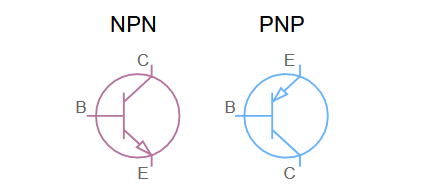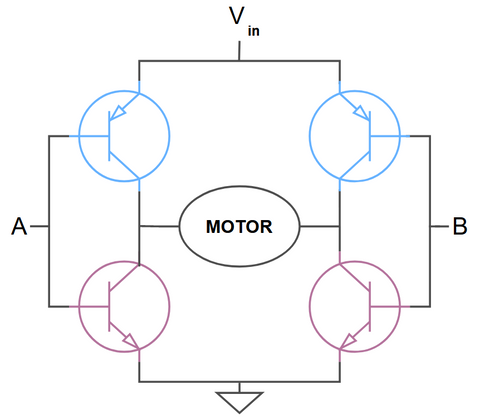Figure 1: Illustration of a circuit for an H-bridge. The H-Bridge is constructed with 4 BJT transistors - two PNP transistors (blue) and two NPN transistors (purple) and a DC motor is located in the middle.

In figure 1 we see that the H-bridge is constructed of two PNP transistors (blue) and two NPN transistors (purple). BJT transistors are used here, as we already have a blog about Analog Circuits that explains a little about how BJT transistors work. As we also mention in the analog circuits blog, BJT and MOSFET transistors perform similar functions. So both MOSFETs g BJTs can be used in H-bridges and the choice of which to use depends on the specific application requirements.

In general, MOSFETs are preferred over BJTs in H-bridges because they have lower on-state resistance, resulting in lower power dissipation and higher efficiency. They also have faster switching speeds and thus lower switching losses (See the blog on Power Electronics! to learn about swithing losses). MOSFETs can also operate at higher frequencies than BJTs.
However, BJTs are some tough fellows and are typically more robust and tolerant of overvoltage conditions, which can be important in some applications. In addition, BJTs are used in low-voltage h-bridge circuits, where swithing losses are typically smaller. BJTs also have relatively low on-resistance and high current gain, making them suitable for controlling small to medium loads. Whats important to note here is that when designing an H-bridge, it is important to consider the trade-off between different transistor types. In our case here, which is to learn about the H-bridge, the BJT transistor works just fine.

In figure 1 we see that the base of the two transistors on the left side of the motor is connected to point A and that the bases of the remaining two transistors are connected to point B. At the bottom of figure 1 we have the emitter of our NPN transistors connected to ground . At the top of the figure, we have our PNP connected to Vin, which is our positive voltage. The trick here is that by changing the voltage across points A and B to HIGH /LOW or 1 and 0, we can change the direction of the current through the motor. There are four different combinations of voltages across points A and B, see Figure 2.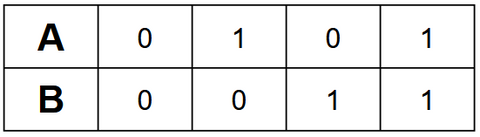Figure 2: Illustration of the four combinations of voltages.

If we take a deep breath and take a sip of coffee, we might notice that if A and B are 0 then their associated PNP transistors will open and let a current flow through them, see figure 3.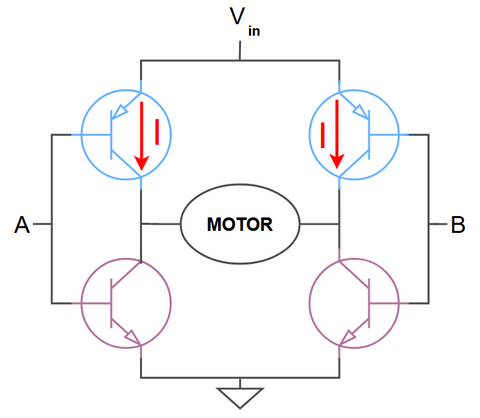Figure 3: Illustration of the circuit when the voltages across A and B are 0.

When A and B are 1, the NPN transistors will be opened, see figure 4.Figure 4: Illustration of the circuit when the voltages across A and B are 1.

But why is it that so good to know? Well, this concept is actually super important because with this setup it's not possible for any current to run directly through the network as shown below in Figure 5.Figure 5: Illustration of a non-possible direction for the current in the network.

This is a good thing because if the current could flow in that direction then we would be dealing with a short circuit and the transistors would most likely break and the DC motor would not be able to work in either direction at all.
So this is a direction we do not want for the current. But which directions are we interested in? As said, if both A and B are 0 then no current will flow as both our NPNs are closed and only our PNPs are open. And as you might have guessed, when both A and B are 1 we have the same principle - no current - but now because our NPNs open and our PNPs close.
This means that these cases will constitute that the DC motor is in an inactive state. This means that there are only two cases in our table in Figure 2 that constitute the drive of the DC motor, see Figure 6.Figure 6: Case of operation for the motor seen in the network in Figure 1.

So it is these two cases in the green circle that we are interested in and each case will drive the motor in the opposite direction in relation to the other.

For the first of the two cases in the green circle, we have that A is 1 and B is 0. The current will then flow from Vin through the PNP transistor on the right at point B, through the motor and on to the output through the NPN transistor at point A, as shown in figure 7.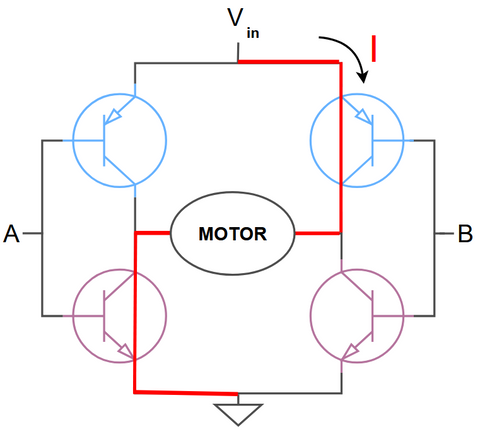Figure 7: Illustrate the path of the current when A is 1 and B is 0.

For the second case in the green circle we have that A is 0 and B is 1. This will therefore allow the current to flow through the motor in the opposite direction than in the previous case illustrated in figure 7. The current will then flow from Vin through the PNP transistor on the left side at point A, through the motor and further towards the output through the NPN transistor at point B, as shown in figure 8.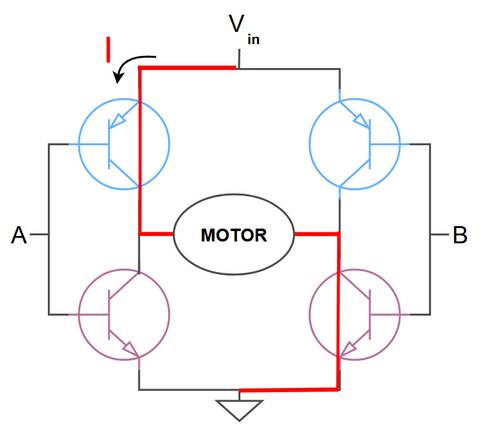Figure 8: Illustrate the path of the current when A is 0 and B is 1.

This is the method by which H-bridges can change the direction and amount of current that is deposited in the motor.

One of the most common applications of H-bridges is in the control of robots and other automated systems where there is a need to control the movement of motors in different directions and speeds. H-bridges can also be used in other applications, such as in solar systems, where they are used to regulate the current and voltage from the solar panels.

In addition, H-bridges have also become popular in DIY electronics and hobby projects, where they can be used to control various electronic devices and motors!

Now we have an understanding of how an H-bridge works, so let's now go through a demonstration of how we can use the H-bridge MX1508 in practice with a microcontroller.

## Demonstration - MX1508

The following circuit shows how to connect an Arduino UNO to the H-bridge MX1508. Connect wires as shown in Figure 9.Figure 9: Circuit for the H-bridge, MX1508, and a DC motor.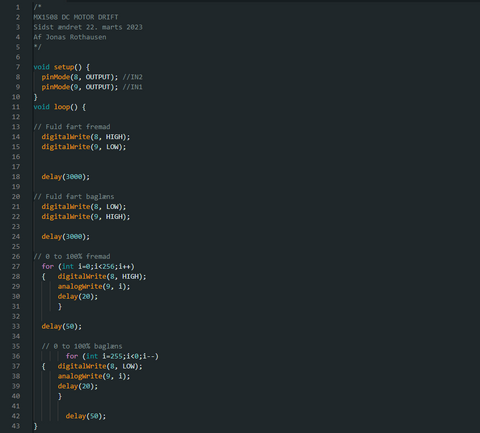Figure 10: Arduino IDE code for DC motor drive in both directions. The code can be found and copied from this GitHub Link.

As you can see in the code, the motor first moves forward for 3 seconds and backwards for 3 seconds. Then the motor stops and its speed increases from 0 to 100% forward with constant acceleration, and then the same movement is performed in reverse.

### Required Components

To make this project you will need the components below. Most components can be found here at eBits.dk.

 Amount Picture Components 1Arduino Uno 1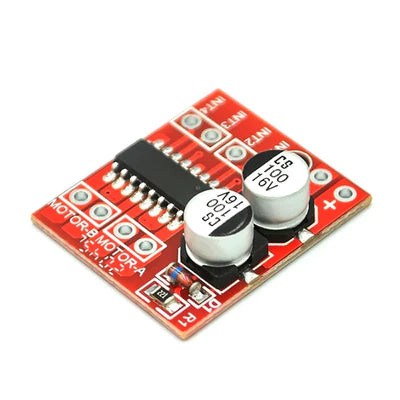MX1508 H-bridge 7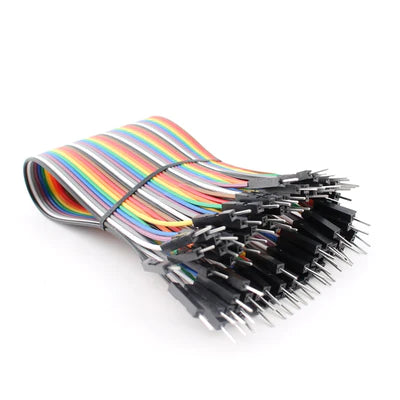Cables(male/male) 1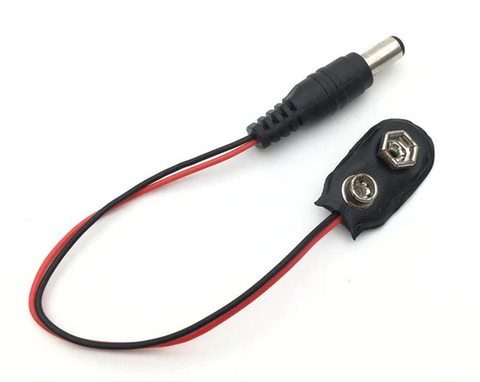9 V Battery 1 DC Motor

Note that microcontrollers other than the Arduino UNO can be used.

1 kommentarKatherine

How much of a delay between A and B should we code in to avoid shoot through?

Skriv kommentar!